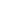Flat 50% off

Ends in

•• Mathematics
• Back to Doubt Clearing

## Please solve this question.If $(\sin \alpha) x^{2}-2 x+b \geq 2$for all real values of $x \leq 1$ and $\alpha \in\left(0, \frac{\pi}{2}\right) \cup\left(\frac{\pi}{2}, \pi\right)$, then the possible real values of b is/are(a) 2(b) 3(c) 4(d) 5

### Asked By Subha Bal Pal

Updated Mon, 20 May 2019 02:56 pm